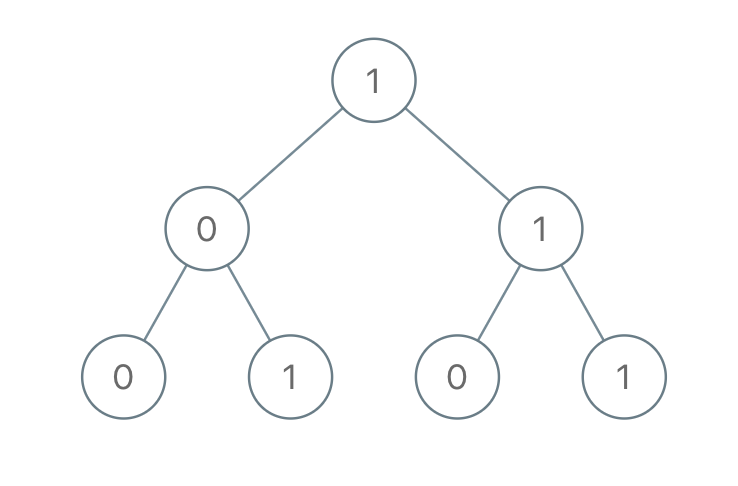## C# || How To Get Total Sum Root To Leaf Binary Numbers In Binary Tree Using C#The following is a module with functions which demonstrates how to get the total sum root to leaf binary numbers in a binary tree using C#.

1. Sum Root To Leaf – Problem Statement

You are given the root of a binary tree where each node has a value 0 or 1. Each root-to-leaf path represents a binary number starting with the most significant bit.

• For example, if the path is 0 -> 1 -> 1 -> 0 -> 1, then this could represent 01101 in binary, which is 13.

For all leaves in the tree, consider the numbers represented by the path from the root to that leaf. Return the sum of these numbers.

The test cases are generated so that the answer fits in a 32-bits integer.

A leaf node is a node with no children.

Example 1:``` Input: root = [1,0,1,0,1,0,1] Output: 22 Explanation: (100) + (101) + (110) + (111) = 4 + 5 + 6 + 7 = 22 ```

Example 2:

``` Input: root =  Output: 0 ```

2. Sum Root To Leaf – Solution

The following is a solution which demonstrates how to get the total sum root to leaf binary numbers in a binary tree.

This solution uses Depth First Search to explore items at each level.

``` 2. Sum Root To Leaf - Solution C# // ============================================================================ // Author: Kenneth Perkins // Date: Jan 10, 2022 // Taken From: http://programmingnotes.org/ // File: Solution.cs // Description: Demonstrates how to get sum root to leaf binary numbers // ============================================================================ /** * Definition for a binary tree node. * public class TreeNode { * public int val; * public TreeNode left; * public TreeNode right; * public TreeNode(int val=0, TreeNode left=null, TreeNode right=null) { * this.val = val; * this.left = left; * this.right = right; * } * } */ public class Solution { public int SumRootToLeaf(TreeNode root) { return Traverse(root, 0); } private int Traverse(TreeNode node, int currentSum) { if (node == null) { return 0; } // Calculate current sum currentSum = (currentSum * 2) + node.val; // We have reached a leaf node if (node.left == null && node.right == null) { return currentSum; } // Keep traversing left and right calculating the sum return Traverse(node.left, currentSum) + Traverse(node.right, currentSum); } }// http://programmingnotes.org/ 123456789101112131415161718192021222324252627282930313233343536373839 // ============================================================================//    Author: Kenneth Perkins//    Date:   Jan 10, 2022//    Taken From: http://programmingnotes.org///    File:  Solution.cs//    Description: Demonstrates how to get sum root to leaf binary numbers// ============================================================================/** * Definition for a binary tree node. * public class TreeNode { *     public int val; *     public TreeNode left; *     public TreeNode right; *     public TreeNode(int val=0, TreeNode left=null, TreeNode right=null) { *         this.val = val; *         this.left = left; *         this.right = right; *     } * } */public class Solution {    public int SumRootToLeaf(TreeNode root) {        return Traverse(root, 0);    }    private int Traverse(TreeNode node, int currentSum) {        if (node == null) {            return 0;        }        // Calculate current sum        currentSum = (currentSum * 2) + node.val;         // We have reached a leaf node        if (node.left == null && node.right == null) {            return currentSum;        }        // Keep traversing left and right calculating the sum        return Traverse(node.left, currentSum) + Traverse(node.right, currentSum);    }}// http://programmingnotes.org/ ```

QUICK NOTES:
The highlighted lines are sections of interest to look out for.

The code is heavily commented, so no further insight is necessary. If you have any questions, feel free to leave a comment below.

Once compiled, you should get this as your output for the example cases:

``` 22 0 ```